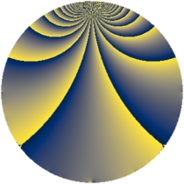# Properties

 Label 92.2.hLevel $92$ Weight $2$ Character orbit 92.h Rep. character $\chi_{92}(7,\cdot)$ Character field $\Q(\zeta_{22})$ Dimension $100$ Newform subspaces $1$ Sturm bound $24$ Trace bound $0$

# Related objects

## Defining parameters

 Level: $$N$$ $$=$$ $$92 = 2^{2} \cdot 23$$ Weight: $$k$$ $$=$$ $$2$$ Character orbit: $$[\chi]$$ $$=$$ 92.h (of order $$22$$ and degree $$10$$) Character conductor: $$\operatorname{cond}(\chi)$$ $$=$$ $$92$$ Character field: $$\Q(\zeta_{22})$$ Newform subspaces: $$1$$ Sturm bound: $$24$$ Trace bound: $$0$$

## Dimensions

The following table gives the dimensions of various subspaces of $$M_{2}(92, [\chi])$$.

Total New Old
Modular forms 140 140 0
Cusp forms 100 100 0
Eisenstein series 40 40 0

## Trace form

 $$100q - 7q^{2} - 11q^{4} - 22q^{5} - 12q^{6} - 10q^{8} - 12q^{9} + O(q^{10})$$ $$100q - 7q^{2} - 11q^{4} - 22q^{5} - 12q^{6} - 10q^{8} - 12q^{9} - 11q^{10} - 18q^{12} - 18q^{13} - 11q^{14} + 5q^{16} - 22q^{17} - 24q^{18} - 11q^{20} - 22q^{21} - 30q^{24} - 16q^{25} + 12q^{26} - 11q^{28} - 42q^{29} - 11q^{30} - 27q^{32} - 22q^{33} + 11q^{34} + 77q^{36} - 22q^{37} + 44q^{38} + 77q^{40} - 10q^{41} + 99q^{42} + 66q^{44} + 65q^{46} + 38q^{48} - 8q^{49} + 30q^{50} + 96q^{52} - 22q^{53} + 48q^{54} + 44q^{56} - 22q^{57} + 79q^{58} + 11q^{60} - 22q^{61} - 36q^{62} + 10q^{64} - 22q^{65} - 44q^{66} - 10q^{69} + 34q^{70} - 21q^{72} - 18q^{73} - 22q^{74} - 66q^{76} + 122q^{77} - 66q^{78} - 110q^{80} + 8q^{81} - 122q^{82} - 165q^{84} + 54q^{85} - 121q^{86} - 99q^{88} + 22q^{89} - 198q^{90} - 86q^{92} + 212q^{93} - 61q^{94} - 147q^{96} + 22q^{97} - 71q^{98} + O(q^{100})$$

## Decomposition of $$S_{2}^{\mathrm{new}}(92, [\chi])$$ into newform subspaces

Label Dim. $$A$$ Field CM Traces $q$-expansion
$$a_2$$ $$a_3$$ $$a_5$$ $$a_7$$
92.2.h.a $$100$$ $$0.735$$ None $$-7$$ $$0$$ $$-22$$ $$0$$